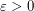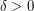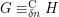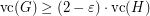# counting quantifiers

## Vertex Cover Integrality Gap ★★

Author(s): Atserias

Conjecture   For everythere issuch that, for every large, there are-vertex graphsandsuch thatand.

Keywords: counting quantifiers; FMT12-LesHouches

## Fixed-point logic with counting ★★

Author(s): Blass

Question   Can either of the following be expressed in fixed-point logic plus counting:
\item Given a graph, does it have a perfect matching, i.e., a setof edges such that every vertex is incident to exactly one edge from? \item Given a square matrix over a finite field (regarded as a structure in the natural way, as described in [BGS02]), what is its determinant?